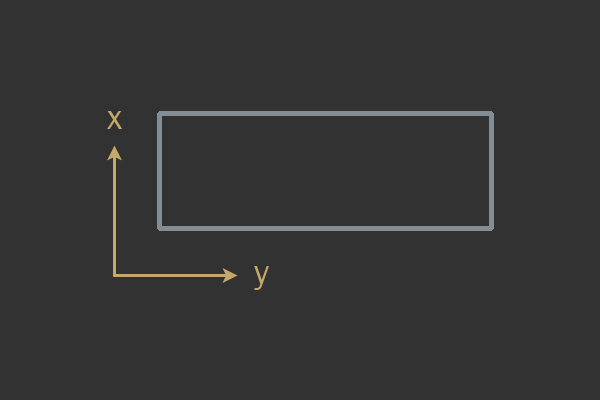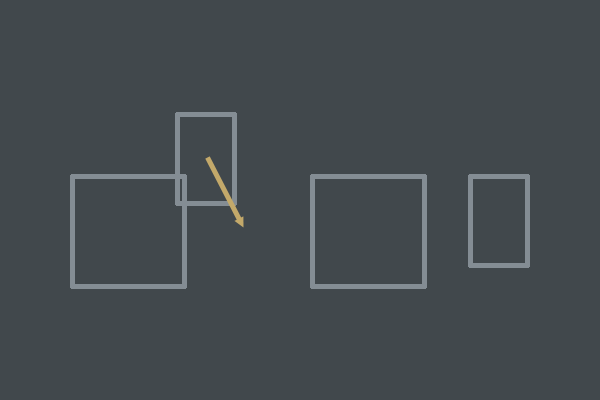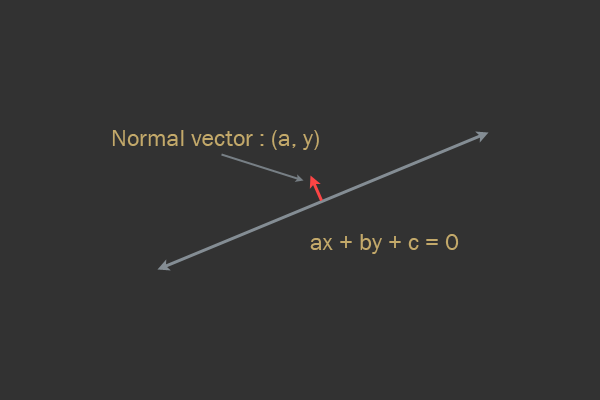# 阅读记录How to Create a Custom 2D Physics Engine - Rangy Gaul（1）

A custom physics engine can tackle any sort of technical effect the creator has the skill to create.自定义物理引擎可以处理创作者有能力创造的任何技术效果。

## The Basics and Impulse Resolution

### Axis Aligned Bounding Boxes

AABB盒为复杂几何体做用于一个简单测试AABB的数据结构：

struct AABB
{
Vec2 min;
Vec2 max;
};


In order to tell whether two AABB shapes are intersecting you will need to have a basic understanding of the Separating Axis Theorem (SAT).

Here's a quick test taken from Real-Time Collision Detection by Christer Ericson, which makes use of the SAT:实时碰撞检测中AABB测试的SAT的算法大致如下

bool AABBvsAABB( AABB a, AABB b )
{
// Exit with no intersection if found separated along an axis
if(a.max.x < b.min.x or a.min.x > b.max.x) return false
if(a.max.y < b.min.y or a.min.y > b.max.y) return false

// No separating axis found, therefor there is at least one overlapping axis
return true
}


### Circle

struct Circle
{
Vec position
};


### 冲量方法Equation 1

$V^{AB} = V^B - V^A$

n用于表示碰撞的法向量

Equation 2

$V^{AB} \cdot n = (V^B - V^A) \cdot n$

Equation 3 点乘式子

$V_1 = \begin{bmatrix}x_1 \\y_1\end{bmatrix}, V_2 = \begin{bmatrix}x_2 \\y_2\end{bmatrix} \\ V_1 \cdot V_2 = x_1 * x_2 + y_2 * y_2$

// Given two objects A and B
e = min( A.restitution, B.restitution )


Equation 4

$V' = e * V$

$V^{'AB} \cdot n = -e * (V^B - V^A) \cdot n$

Equation 6

$V' = V + j * n$

Equation 7

$Impulse = mass * Velocity \\ Velocity = \frac{Impulse}{mass} \therefore V' = V + \frac{j * n}{mass}$

Equation 8 (这里的正负号也有修改，我是觉得碰撞A的话用减更好，符号和碰撞向量的定义有关，取正负我觉得都可以，这里取负主要是为了和后面的推导过程不冲突)

$V'^A = V^A - \frac{j * n}{mass^A} \\ V'^B = V^B + \frac{j * n}{mass^B}$

Equation 9

$(V^B - V^A + \frac{j * n}{mass^A} + \frac{j * n}{mass^B}) * n = -e * (V^B - V^A) \cdot n \\ \therefore \\ (V^B - V^A + \frac{j * n}{mass^A} + \frac{j * n}{mass^B}) * n + e * (V^B - V^A) \cdot n = 0$

Equation 10

$(V^B - V^A) \cdot n + j * (\frac{n}{mass^A} + \frac{n}{mass^B}) * n + e * (V^B - V^A) \cdot n = 0 \\ \therefore \\ (1 + e)((V^B - V^A) \cdot n) + j * (\frac{ n}{mass^A} + \frac{ n}{mass^B}) * n = 0 \\ \therefore \\ j = \frac{-(1 + e)((V^B - V^A) \cdot n)}{\frac{1}{mass^A} + \frac{1}{mass^B}}$

n是方向向量，单位向量，自乘就等于1

void ResolveCollision( Object A, Object B )
{
// Calculate relative velocity
Vec2 rv = B.velocity - A.velocity

// Calculate relative velocity in terms of the normal direction
float velAlongNormal = DotProduct( rv, normal )

// Do not resolve if velocities are separating
if(velAlongNormal > 0)
return;

// Calculate restitution
float e = min( A.restitution, B.restitution)

// Calculate impulse scalar
float j = -(1 + e) * velAlongNormal
j /= 1 / A.mass + 1 / B.mass

// Apply impulse
Vec2 impulse = j * normal
A.velocity -= 1 / A.mass * impulse
B.velocity += 1 / B.mass * impulse
}A.inv_mass = 1 / A.mass


float mass_sum = A.mass + B.mass
float ratio = A.mass / mass_sum
A.velocity -= ratio * impulse

ratio = B.mass / mass_sum
B.velocity += ratio * impulse


### Sinking Object问题

if(A.mass == 0)
A.inv_mass = 0
else
A.inv_mass = 1 / A.mass


void PositionalCorrection( Object A, Object B )
{
const float percent = 0.2 // usually 20% to 80%
Vec2 correction = penetrationDepth / (A.inv_mass + B.inv_mass)) * percent * n
A.position -= A.inv_mass * correction
B.position += B.inv_mass * correction



void PositionalCorrection( Object A, Object B )
{
const float percent = 0.2 // usually 20% to 80%
const float slop = 0.01 // usually 0.01 to 0.1
Vec2 correction = max( penetration - k_slop, 0.0f ) / (A.inv_mass + B.inv_mass)) * percent * n
A.position -= A.inv_mass * correction
B.position += B.inv_mass * correction
}


### 简单流型生成

struct Manifold
{
Object *A;
Object *B;
float penetration;
Vec2 normal;
};


## Core Engine

### 积分

F = ma

// Explicit Euler
x += v * dt
v += (1/m * F) * dt


dt表示delta time

// Symplectic Euler
v += (1/m * F) * dt
x += v * dt


Using the exact same dt value in your code everywhere will actually make your physics engine deterministic, and is known as a fixed timestep

const float fps = 100
const float dt = 1 / fps
float accumulator = 0

// In units of seconds
float frameStart = GetCurrentTime( )

// main loop
while(true)
const float currentTime = GetCurrentTime( )

// Store the time elapsed since the last frame began
accumulator += currentTime - frameStart( )

// Record the starting of this frame
frameStart = currentTime

while(accumulator > dt)
UpdatePhysics( dt )
accumulator -= dt

RenderGame( )


Chunks of dt are removed from the accumulator until the accumulator is smaller than a dt chunk.

// Avoid spiral of death and clamp dt, thus clamping
// how many times the UpdatePhysics can be called in
// a single game loop.
if(accumulator > 0.2f)
accumulator = 0.2f


const float fps = 100
const float dt = 1 / fps
float accumulator = 0

// In units seconds
float frameStart = GetCurrentTime( )

// main loop
while(true)
const float currentTime = GetCurrentTime( )

// Store the time elapsed since the last frame began
accumulator += currentTime - frameStart( )

// Record the starting of this frame
frameStart = currentTime

// Avoid spiral of death and clamp dt, thus clamping
// how many times the UpdatePhysics can be called in
// a single game loop.
if(accumulator > 0.2f)
accumulator = 0.2f

while(accumulator > dt)
UpdatePhysics( dt )
accumulator -= dt

const float alpha = accumulator / dt;

RenderGame( alpha )

void RenderGame( float alpha )
for shape in game do
// calculate an interpolated transform for rendering
Transform i = shape.previous * alpha + shape.current * (1.0f - alpha)
shape.previous = shape.current
shape.Render( i )


1、这样需要预测

2、物理引擎中突变的情况很多，对于这种突变的预测产生的错误预测往往会导致一些瞬移

### 模块设计

Bodies 物理主体是一个包含了关于某个给定物理对象的所有信息的对象。它将存储对象所代表的形状、大量数据、变换(位置、旋转)、速度、扭矩等。

struct body
{
Shape *shape;
Transform tx;
Material material;
MassData mass_data;
Vec2 velocity;
Vec2 force;
real gravityScale;
};


body应该有多种Shape，以组合的方式提供，可以添加多种shape

struct MassData
{
float mass;
float inv_mass;

float inertia;
float inverse_inertia;
};


### 宽相位计算

struct Pair
{
body *A;
body *B;
};


// Generates the pair list.
// All previous pairs are cleared when this function is called.
{
pairs.clear( )

// Cache space for AABBs to be used in computation
// of each shape's bounding box
AABB A_aabb
AABB B_aabb

for(i = bodies.begin( ); i != bodies.end( ); i = i->next)
{
for(j = bodies.begin( ); j != bodies.end( ); j = j->next)
{
Body *A = &i->GetData( )
Body *B = &j->GetData( )

// Skip check with self
if(A == B)
continue

A->ComputeAABB( &A_aabb )
B->ComputeAABB( &B_aabb )

if(AABBtoAABB( A_aabb, B_aabb ))
pairs.push_back( A, B )
}
}
}


// Sort pairs to expose duplicates
sort( pairs, pairs.end( ), SortPairs );

// Queue manifolds for solving
{
int i = 0;
while(i < pairs.size( ))
{
Pair *pair = pairs.begin( ) + i;
uniquePairs.push_front( pair );

++i;

// Skip duplicate pairs by iterating i until we find a unique pair
while(i < pairs.size( ))
{
Pair *potential_dup = pairs + i;
if(pair->A != potential_dup->B || pair->B != potential_dup->A)
break;
++i;
}
}
}
bool SortPairs( Pair lhs, Pair rhs )
{
if(lhs.A < rhs.A)
return true;

if(lhs.A == rhs.A)
return lhs.B < rhs.B;

return false;
}


### 分层

// Generates the pair list.
// All previous pairs are cleared when this function is called.
{
pairs.clear( )

// Cache space for AABBs to be used in computation
// of each shape's bounding box
AABB A_aabb
AABB B_aabb

for(i = bodies.begin( ); i != bodies.end( ); i = i->next)
{
for(j = bodies.begin( ); j != bodies.end( ); j = j->next)
{
Body *A = &i->GetData( )
Body *B = &j->GetData( )

// Skip check with self
if(A == B)
continue

// Only matching layers will be considered
if(!(A->layers & B->layers))
continue;

A->ComputeAABB( &A_aabb )
B->ComputeAABB( &B_aabb )

if(AABBtoAABB( A_aabb, B_aabb ))
pairs.push_back( A, B )
}
}
}


### Halfspace Intersection

$General \ form:ax+by+c=0\\ Normal\ to\ line:\begin{bmatrix} a\\b \end{bmatrix}$2021.8.25

posted @ 2021-08-25 11:19  飞翔的子明  阅读(148)  评论(0编辑  收藏  举报